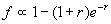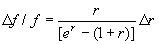# IV. 2MASS Data Processing

## 5. Extended Source Identification and Photometry

### f. Galaxy Photometry Error Tree

The fundamental limits to 2MASS galaxy photometry are set by the accuracy to which the background level can be determined, by the total signal to noise ratio in the aperture used to report the flux of a galaxy, and by the zero-point calibration used to adjust raw magnitudes onto a standard scale. Most of the time, the dominant error is the Poisson noise due to the background summed over the pixels used to make the photometric measurement and the uncertainty in the standard background removal itself (see IV.5a). However, a significant portion of the 2MASS data is affected by high-frequency background variation, which causes the photometric error to be larger for a small fraction of the galaxies. There are two sources for such background variations, one being atmospheric "airglow" and the other camera hardware related. In the near-infrared, the background levels generally fluctuate due to airglow emission, sometimes at very high spatial frequencies, particularly at 1.6 µm. In addition to airglow, 2MASS images include electronic ("pickup") noise that is correlated and can, at times, be comparable to the airglow component. Electronic pickup refers to image features that are not of astronomical origin, but instead are associated with the camera electronics. These features may manifest as periodic horizontal stripes or abrupt jumps in the background level. The impact is mostly felt in the galaxy photometry, where a systematic bias is introduced. This bias can be as large as 20% in some cases, but is more typically 3 to 7% (usually an overestimate of the galaxy flux). Examples of both forms of background enhancement are given in Figure 29.

For the usual case where the galaxy flux is negligible compared to the background flux, the measurement error in summing up the flux over n pixels in the Atlas image is(Eq. IV.5.9)

wherepix is the measured pixel noise as measured using an entire Atlas image (512×1024 pixels). The factor of 1.7 accounts for the smoothing introduced in the Atlas image by the frame resampling and construction process, and the factor of 4 results from the correlation of the flux in 1´´ pixels, due to the 2´´ camera (raw frame).

GALWORKS also models and subtracts variations in the background using a polynomial fitting scheme described IV.5a. If the background variations are small on time scales of a few seconds, the fitting procedure is limited primarily by the effective RMS in the background sky noise,pix. The fitting procedure usually can match the background over regions as small as ~2´ to 3´. Empirical tests indicate that the background fitting procedure is accurate to within 3 to 5% of the RMS pixel noise (in the absence of abrupt background structure coming from high-frequency airglow or electronic banding, which is usually the case for the 2.2µm images). Since errors in the background contribute systematically to all pixels in a galaxy smaller than 3´, this error contributes in proportion to the number of pixels n. The total photometric noise variance is the quadratic sum of these two terms(Eq. IV.5.10)

It is clear that the first term dominates the total error except when the number of pixels within the aperture is very large, n > 104 (corresponding to a circular radius > 40 to 50 pixels). This also assumes that background variation is well behaved. Most galaxies detected in 2MASS are small and faint, so the Poisson noise component (Eq. IV.5.9) should dominate the total photometric error. Consider for example a Ks=13 mag galaxy. The integrated flux is ~630 DN (or ~4.5 mJy) using a typical Ks zero point magnitude of 20.0 mag (relating the calibrated mag to the raw or "data number" flux; see Eq. IV.5.8). The typical size for a galaxy of this brightness is about 16´´ in diameter, corresponding to a circular aperture with ~200 pixels. The typical Ks background pixel noise is ~1 DN (or 20 mag per arcsec2). Using Eq. IV.5.10 and the integrated flux of the source, we compute a SNR of 12.6 to 12.8, where the Poisson component is 25 to 70 times larger than the background-fit uncertainty (i.e., the noise contribution from the background fitting procedure is very small, only 1 to 4% of the total photometric noise). For larger (and hence less common) galaxies, the size of the galaxy must be greater then ~35´´ in diameter for the uncertainty in the background fit to become appreciable (>10% of the total).

If the background variation requires a higher-power polynomial than a cubic, then the error in the background will no longer be determined by the pixel noise. Instead, the background error will result from the residual error after a cubic polynomial is fit. This will be the case for severe airglow variation, which can modulate on high spatial frequency scales (see left panel, Figure 29), and from correlated "electronic" noise (see right panel, Figure 29). Both conditions can easily boost the total photometric noise by >50%. Unfortunately, the 2MASS data reduction pipeline is not adequately designed to quantify these severe background variations; and indeed, high-frequency background variations may go undetected from scan to scan. We are exploring methods at which detection and correction of severe 'airglow features" may be possible, particularly for the H-band data. Fortunately, the hardware-induce background variations are diminishing with maturity of the 2MASS survey.

Adaptive apertures (i.e., Kron and isophotal) come in two different shapes: circular and elliptical. Circular apertures are fully described by the radius, fit to the desired isophote. Elliptical apertures are described by three parameters, radius (semi-major axis), axis ratio and position angle, fit to the desired isophote. The fitting procedures introduce additional uncertainties. Here we assume the uncertainty due to the position of the isophote is negligible.

For the circular case, the radius has an error that is driven by background noise and contamination (i.e., stars near or within the isophote). Errors in the determination of that radius dominate the photometric error tree. Consider the typical case of a galaxy with an exponential profile. The integral flux over a circular aperture is(Eq. IV.5.11)

where r is the aperture radius divided by the radial scale length of the galaxy profile. The flux error caused by the error in radius determination is(Eq. IV.5.12)

For a disk scale length of 2´´, and typical isophotal 20 mag arcsec-2 aperture radii of 6 to 8´´, the flux error ranges between 0.08 and 0.19 ×r. The typical width or uncertainty of the circular isophote is about 1´´, sor=0.5 and the resultant flux error ranges between 4-9%. The Poisson photon noise in an aperture of this size gives typical flux errors of ~5-7%, and hence the radius uncertainty is comparable to the photon-noise contribution for this Ks~13 mag spiral galaxy case.

For elliptical aperture photometry, in addition to the radial uncertainty, two additional parameters (axial ratio and position angle) add to the total error budget. However, there is some positive compensation due to the fact that the orientation of galaxies is elliptically shaped; thus, optimal elliptical apertures minimize the background noise contribution and systematics due to measurement error in the background itself (above). Analysis of the 2MASS extended source database reveals that for 2MASS galaxies brighter than ~13 mag, the elliptical aperture photometry gives the most precise measurement of the galaxy flux. Note however that the elliptical aperture is vulnerable to contamination, especially in high source density regions, which makes it susceptible to large uncertainties if the ellipse parameters are inaccurate. For faint galaxies, the PSF shape and image resolution generally favor a circular aperture to that of an ellipse because the galaxies are so small that the ellipse parameters are highly uncertain.

Characterization of the uncertainties due to the background removal process are further discussed in the 2MASS Large Galaxy Atlas (Jarrett et al. 2003, AJ, 125, 525).

[Last Updated: 2003 Mar 10; by T. Jarrett, T. Chester, S. Schneider, S. Van Dyk, & R. Cutri]